Nasca Monkey   Articles      Previous   Cone animation

The Seal of Atlantis

The Cone & Square formation is the essence of the Seal of Atlantis. What practical fields might this blueprint relate to?
Why was the Cone & Square design chosen above others, and repeated at Nasca?  It would seem that to earn such honor, it must be very special.

Geometrical Modules in the Torso

Most of the lines within the Torso hold significant angles with the Square's diagonals x, y. I found this out in an experiment, which I had held back on until I had the torso highly magnified, like in the diagram below These lines together with the Square's diagonals create higher level order. It is a module, which is valid by itself at any scale, but here it is on the same scale with the Square, i.e., it integrates into the Square's system!

The lines c and a subtend the engraved lines c and a, which are irregularly curved. Their exact curvature would be hard to determine, whereas drawing the subtending lines is straightforward. In contrast, the straight lines e, b, and d pass entirely within the engraved originals.
One could object that lines c and a are too hypothetical, but evidently, I had made the translation before knowing how it would turn out. The translation is entirely based on the picture.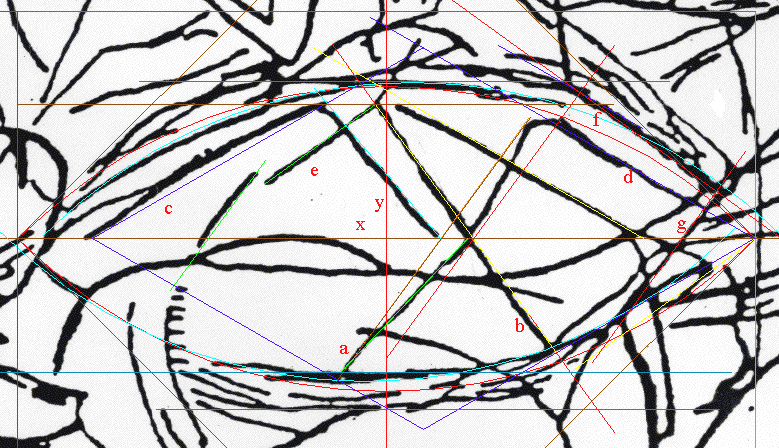From left to right: Line c holds a 30 degree angle with the x-axis. Line e holds a 36 degree angle with the x-axis. Line a holds a 36 degree angle with the y-axis Line b holds a 36 degree angle with the y-axis. Line d holds a 30 degree angle with the y-axis. Line f holds a  36 degree angle with the x-axis Lines a and b create a triangle with the Square's axes, which we find on a regular 5-pointed star (36x36x108 degrees).  This triangle is an example of simple order. That the x-axis does the Golden Section on the vertical side of the triangle is still higher order.I had used compasses to develop the triangle into a 5-pointed star, utilizing the blue circle from diag. 19 below. One of the star's corners falls into the center of the Square!  Then, the lines c, and d join the system of the Square as seen from  the point F (diag 19). F is the focus point of three lines and one circle, four objects altogether. This is enough data to reconstruct the position in any given square..........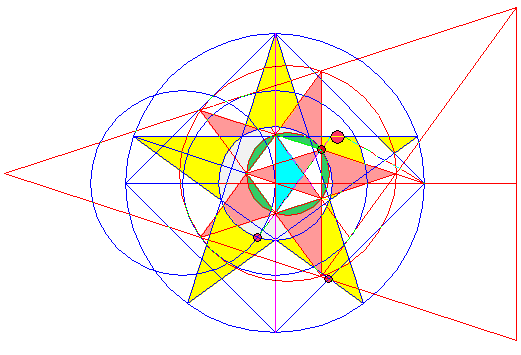The new star, the Pyrostar,  proves to have sprung from the Square. One side of the star's pentagon extends to the 'D' corner of the square. Here, we are solidly on track of the Golden Section in the actual context of our Square. This pentagonal side of the Pyrostar coincides with the 36 degree angle constructed from 'D', and is a tangent to the so called Golden circle centered in the opposite corner of the same square (see below).         The Pyrostar, and the Square's minor Golden Rectangle  This Golden Rectangle (actually white in the diagram) has the length of the Square's diagonal, and the height of the Golden circle's diameter. It is safe to say that it fits the engraving beautifully, see the second diagram down.  Below,  three Pyrostar lines - two of which are the outside pentagon sides - are tangents to Golden circles in diagonally opposite corners of the Square.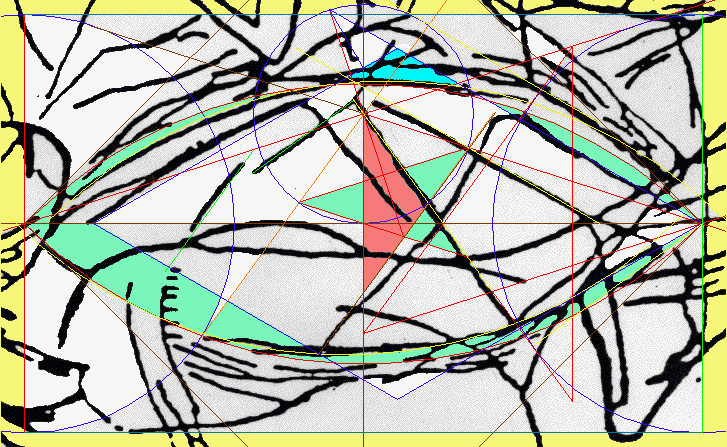The big circle in the diagram below divides the horizontal diagonal in the Phi ratio, and gets us the radius of the Golden Circle, It is centered at the 3/4 point of the square's horizontal diagonal, and passes through two corners of the square.  segment 00 - R  /  seg. G - 00  =  seg. G - 00  /  seg. L - G  =  1.6180339887..  =  Phi The torso's width is well contained within the band of the Golden Rectangle's width (the height of the Golden circle). This is clearly visible.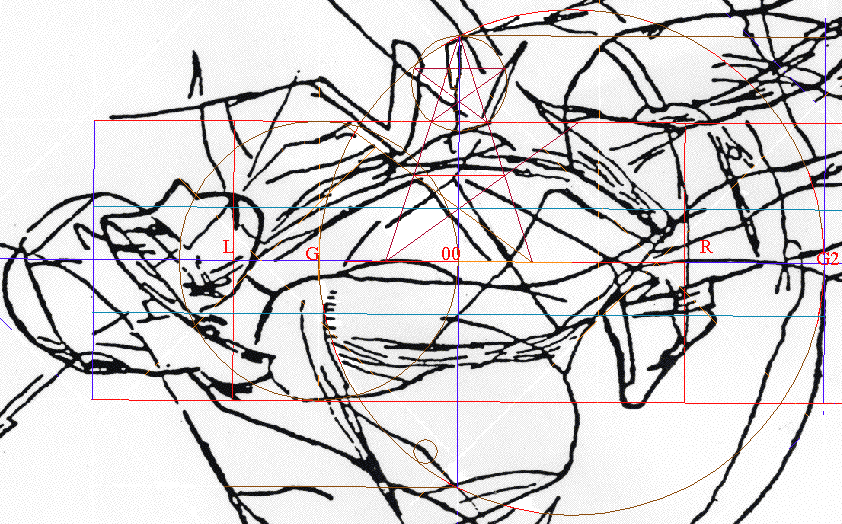Above we can see a strong indication by the engravers of the Golden Section in process within the Square. The big circle is one of the four such possible circles (one for each quadrant), and it definitely fits the engraving, in at least 12-points. We refer to it as the 12-point  circle. Concentrical with the 12-point circle, is the small brown circle in the diagram below. Its diameter forms the Golden ratio with the diameter of the Golden circle itself, as seen from the star in the diagram above. Like the 12-point circle, this small circle also fits the image neatly.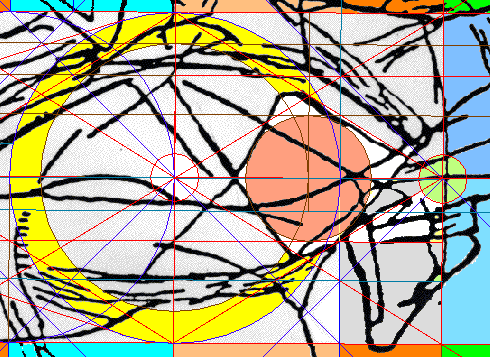It is wedged between the lines a and b (which create the Pyrostar), and lines d and g. So far, the engraved line 'd' translates into a straight line passing entirely within the engraved line. But,  one diagonal of a Golden  Rectangle originating from the point D of the square, subtends the engraved line 'd' with good accuracy. An engraved line of the Torso is symmetrical with the line 'd' across the x-axis.  So, the enclosure of the small brown Golden circle is consistency itself. One  Golden circle and four lines, each having to do with the Golden Section within the Square.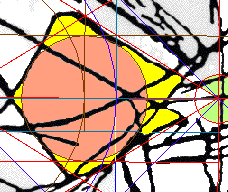The Square's major Golden Rectangle   This is the Golden Rectangle, whose height equals the diagonal of the Square.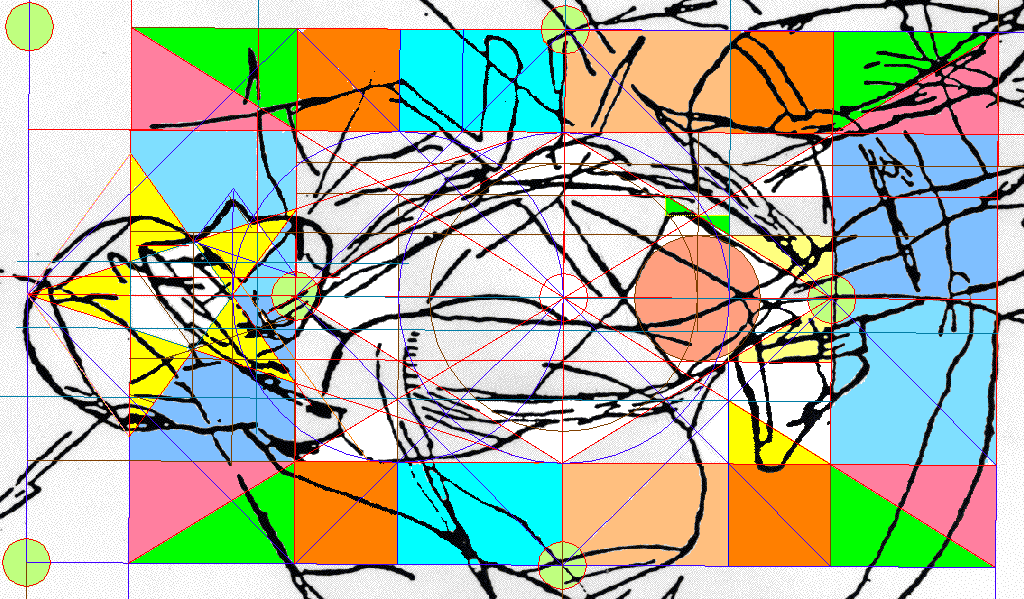Naturally, we want to see the Golden circle with an inscribed  5-pointed star,  which is centered in the left hand corner of the Main Square, because it falls over the head. Please note:  *  The engraved lines fanning out from the forehead coincide with the star.  *  An arm of the same star also limits the chin. *  The same line, including the star's lower right tip,  merges with an engraved line.  *  The base of the star's pentagon limits the width of the head from below.  And, there are still more ways, in which the star inscribed within the Golden circle fits the engraving! The reader can verify this fact independently.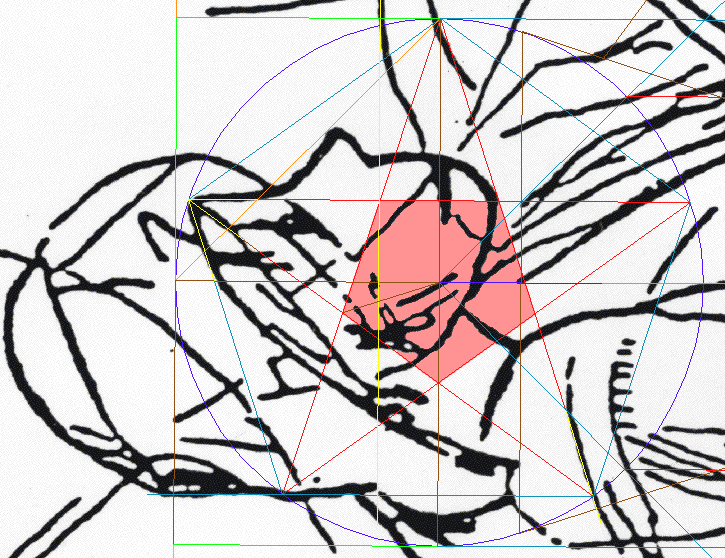There is another excellent example on how the head of the engraving's human figure correlates with the Golden Sectin in the Square,  as well as the Golden circle.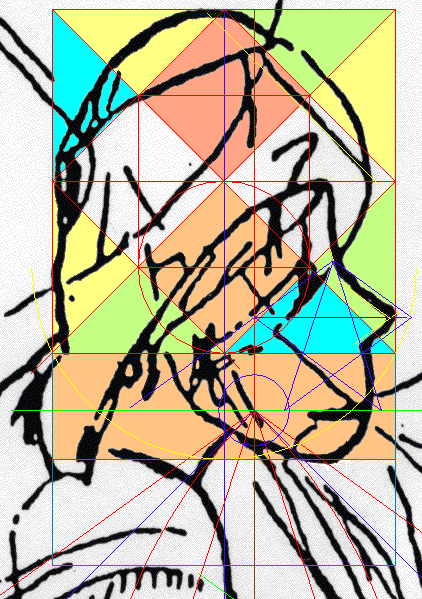Have we seen enough proof of the Square? Although, art-wise, the Athena engraving compares best to a mandala, with no set down, sideways, or up, the Square's X,Y diagonals set the Engraving's main axes, and the x-axis sets the horizon for the engraving's most important view.  The proof of the Square is also in the sheer quantity of subsystems, which  can only originate from it. Here is another such subsystem: *  K-x stars    Like the Pyrostar, the K-x stars exist within the context                      of the Main Square, and are complementary to the Pyrostar                      and to the K-circle of the Cone. The Ultimate Test of the Square Have we seen enough proof of the Square? We certainly saw a lot of it, but the ultimate test is still to come. Consider these facts: * I claim to have learned an entire geometrical system from the engraving. * And I claim that the perimeter of the engraving has segment lengths,  whose values make sense with respect to Phi, Pi, and the Osiris Numbers. * The so called Unit Circle is part of the engraving's geometric system. So, the measurement units of the perimeter (the Frame)  should be directly proportional to the unit circles, right? Yet, in my original exploration on paper, the diameter of the Unit Circle measured as 81 Frame millimeters. These units were therefore not directly proportional with each other. Did the picture's order end there? Another puzzling nuisance was that the Cone and hence its unit circles also seemed slightly too large for the Main Square. At the time, I was already getting used to seeing the engraving's order always win over chaos. There was still hope, because some parts of the design seemed very accurately positioned with respect to the Square. My hypothesis was that since the Square took over from the Cone most of the new geometrical creation, it was also possible to let the Cone itself undergo changes, because the original Cone could always be recreated from the  highly precise Square.  So, presumably, if we imported a bitmap of the engraving into CAD, and inserted  the geometrical system over the Main Square, then the unit circles of the Cone should work out directly proportional to the units of the engraving's perimeter. The diameter of the unit circle should work out to 80 millimeters of the Frame. The Test  With the engraving's bitmap imported into a CAD drawing,  I took  great care to recreate the Square. There seems to be only one distinct way to fit the ideal square over the bitmap, so that all four corners fall upon an engraved part.. Then the rest of the system was inserted over the Square. With the unit circle's diameter set to 80, it was time to remeasure the engraving's Frame.  I am happy to announce that the test was a success. The total perimeter worked out to the same original value of 1226, as it should. Only two segments were off by 1. These errors cancelled each other out. The other segments' values duplicate the original. Check this page soon for more material

Top   Next   Nasca Monkey Report    Articles